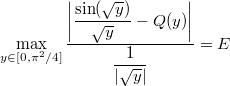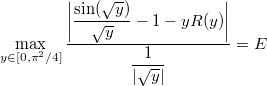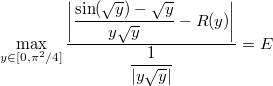Version 1 (modified by sam, 9 years ago) (diff)

--

# Remez tutorial 4/5: fixing lower-order parameters

In the previous section, we took advantage of the symmetry of sin(x) to build the following minimax expression:Leading to the following coefficient, amongst others:

const double a1 = 9.999999766327004083636926544075452569800e-1;


This is an interesting value, because it is very close to 1. Many CPUs can load the value 1 very quickly, which can be a potential runtime gain.

## The brutal way

Now we may wonder: what would be the cost of using 1 here? Let’s see the error value:## The clever way

The clever way involves some more maths. Instead of looking for polynomial Q(y) and setting Q(0) = 1 manually, we search instead for R(y) where Q(y) = 1 + yR(y):Dividing by y up and down gives:Once again, we get a form suitable for the Remez algorithm.

## Source code

#include "lol/math/real.h"
#include "lol/math/remez.h"

using lol::real;
using lol::RemezSolver;

real f(real const &y)
{
real sqrty = sqrt(y);
return (sin(sqrty) - sqrty) / (y * sqrty);
}

real g(real const &y)
{
return re(y * sqrt(y));
}

int main(int argc, char **argv)
{
RemezSolver<3, real> solver;
solver.Run("1e-1000", real::R_PI_2 * real::R_PI_2, f, g, 30);
return 0;
}


Only f and g changed here, as well as the polynomial degree. The rest is the same as in the previous section.

Build and run the above code:

make
./remez


After all the iterations the output should be as follows:

Final error: 4.613764035583927492630791542066631050746e-9
Polynomial estimate:
x**0*-1.666665708792613295297026208726412532085e-1
+x**1*8.333017134192478312472752663154642556843e-3
+x**2*-1.980660618990173078599247774582918018345e-4
+x**3*2.600038299369332084157603784882057661080e-6


We can therefore write the corresponding C++ function:

double fastsin(double x)
{
const double a3 = -1.666665708792613295297026208726412532085e-1
const double a5 = 8.333017134192478312472752663154642556843e-3
const double a7 = -1.980660618990173078599247774582918018345e-4
const double a9 = 2.600038299369332084157603784882057661080e-6

return x + x*x*x * (a3 + x*x * (a5 + x*x * (a7 + x*x * a9))));
}


Note that because of our change of variables, the polynomial coefficients are now a3, a5, a7

## Analysing the results

Let’s see the new error curve:Excellent! The loss of precision is clearly not as bad as before.